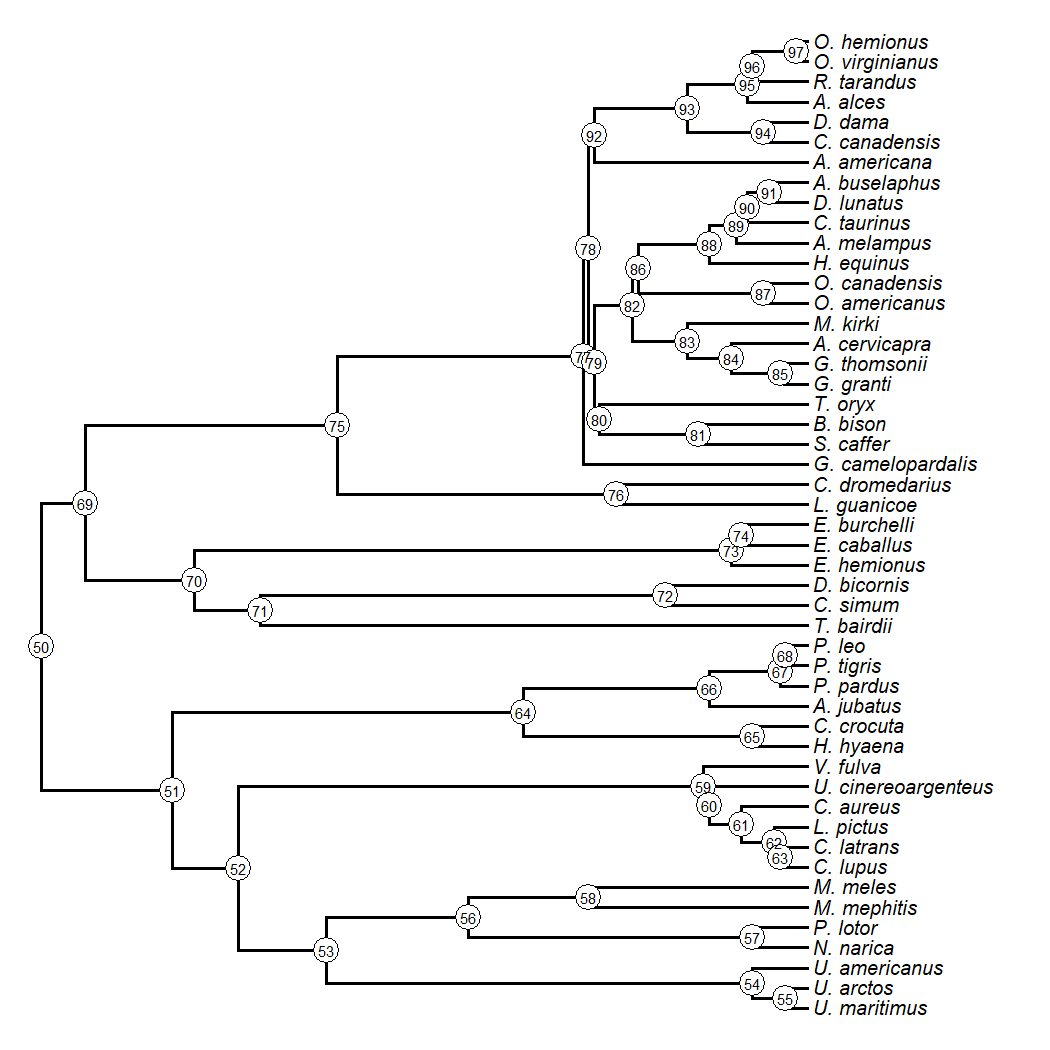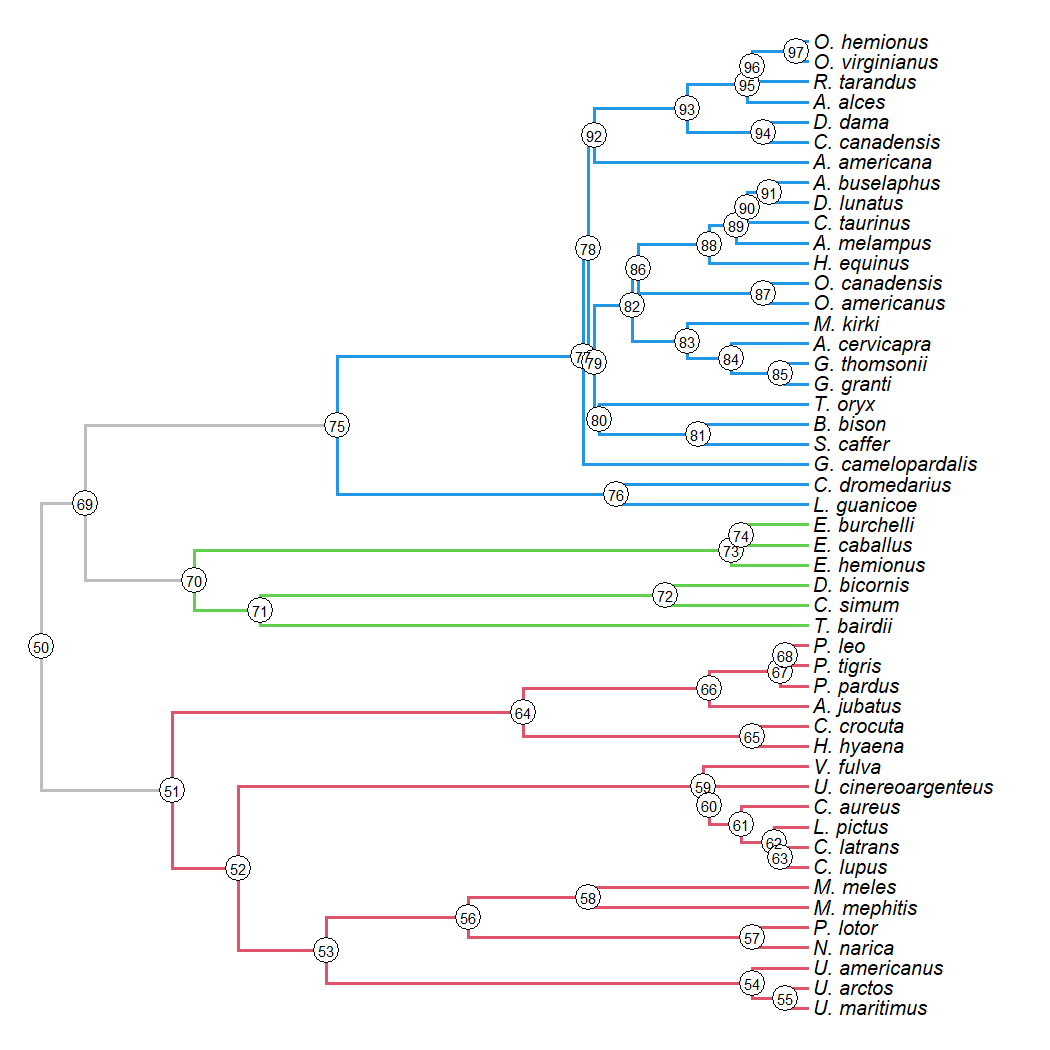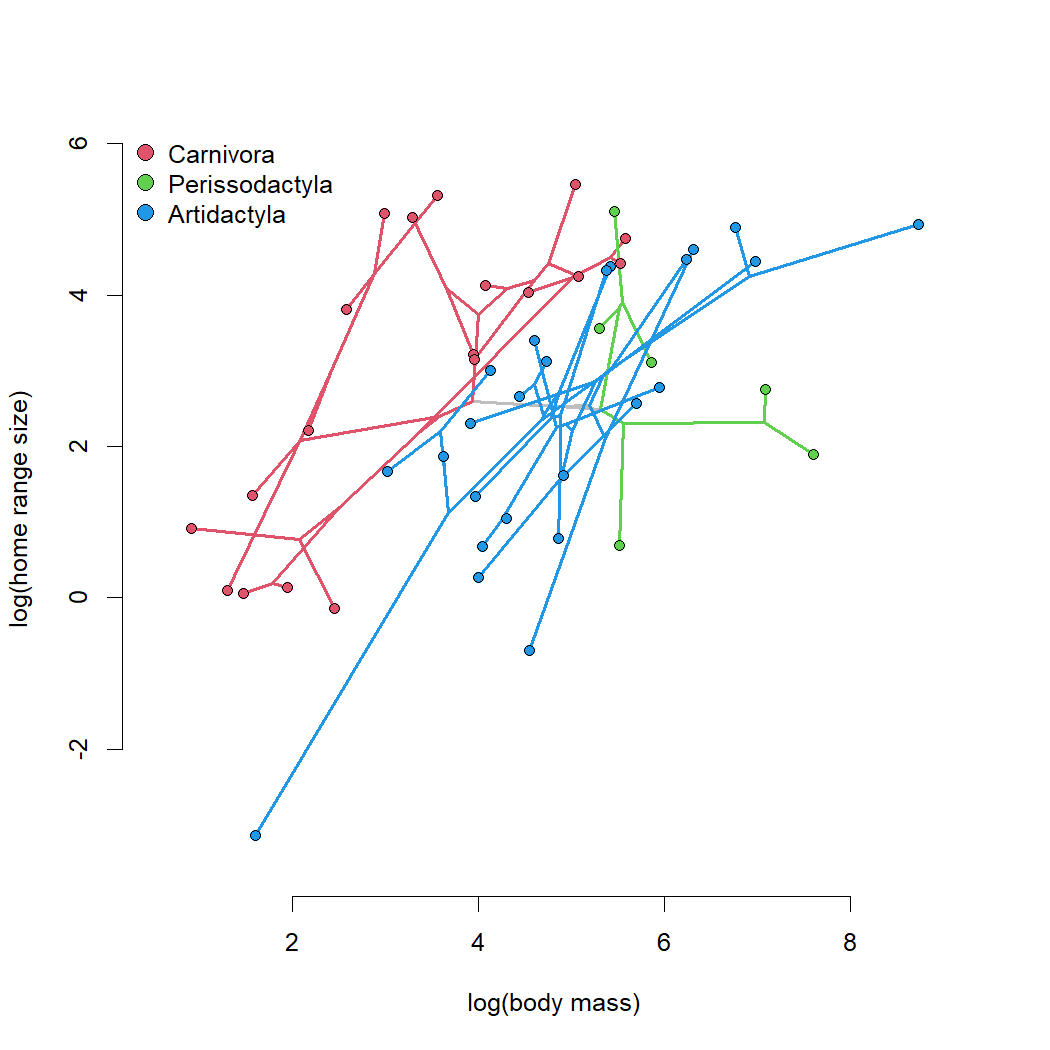## Sunday, November 7, 2021

### Showing different orders or other taxonomic groups in different colors in a phylomorphospace plot

A few days ago, I received the following email about the phytools function `phylomorphospace`:

“I'm trying to make a phylomorphospace that shows me the Orders form the birds in different colors. I tried to follow the example of the sunfishes, where species are in red or in blue depending on the feeding mode. However, I do not know what is happening with my data. I'm sure that the Order column is a factor (with 21 different levels) and one color has been assigned to each level, but I don't know why the phylomorphospace is only showing one color (black). Is there a problem with the color argument in the phylomorphospace function?”

The quick answer to this is that if we want to use colors to show different groups or clades on our tree, we should first use `paintSubTree` to map these groups onto the phylogeny, and then we can use `phylomorphospace` to graph them.

To demonstrate this, I'll use the classic Garland et al. (1992) that comes loaded with the phytools package.

This consists of a phylogeny of mammals, along with a dataset for body mass and home range size for the different species in the tree. Conveniently, this phylogeny only includes representatives of three different mammalian orders (Carnivora, Perissodactyla, and Artiodactyla) so it should be pretty easy to map these three different orders on the tree.

First let's load our packages, tree, and dataset.

``````library(phytools)
data(mammal.tree)
data(mammal.data)
``````

Now let's plot the tree as follows:

``````plotTree(mammal.tree,ftype="i",fsize=0.8)
nodelabels(cex=0.6,frame="circle",bg="white")
``````Maybe we can see from this plot that node 51 corresponds to the most recent common ancestor of all carnivorans, while the Perissodactyla and Artiodactyla are delineated by nodes 70 and 75 respectively. For a larger phylogenies with more orders we could use the function `findMRCA` to identify the MRCA based on a simple list of the members of each clade.

``````nodes<-c(51,70,75)
``````

Next, let's map each of these three clades onto the tree using `paintSubTree`. `paintSubTree` will create an object of a new class: `"simmap"`. In this case, however, we're not using this object type to show the stochastic history of a mapped discrete trait, but the known groups of the terminal taxa in the tree.

``````mammal.orders<-mammal.tree
for(i in 1:length(nodes)){
mammal.orders<-paintSubTree(mammal.orders,node=nodes[i],
stem=FALSE)
}
``````

Now we're ready to plot our tree to verify that we have correctly mapped our clades to each node.

``````plot(mammal.orders,colors<-setNames(c("grey",
nodelabels(cex=0.6,frame="circle",bg="white")
``````Terrific. This is exactly what we were going for.

Lastly, we plot our phylomorphospace as follows:

``````phylomorphospace(mammal.orders,log(mammal.data),colors=colors,
ftype="off",bty="n",node.size=c(0,1),node.by.map=TRUE,
xlab="log(body mass)",ylab="log(home range size)")
legend("topleft",names(colors)[2:4],pch=21,pt.bg=colors[2:4],
bty="n",pt.cex=1.5)
``````#### 1 comment:

1.Tnak you so much, it's very useful. Now it's time to work with own data and PCA's! :D

Note: due to the very large amount of spam, all comments are now automatically submitted for moderation.